# Using musica package

#### 2016-09-03

library(data.table)
library(ggplot2)
library(lubridate)
##
## Attaching package: 'lubridate'
## The following objects are masked from 'package:data.table':
##
##     hour, mday, month, quarter, wday, week, yday, year
## The following object is masked from 'package:base':
##
##     date

### Introduction

The package provides functions for flexible assessment of climate model bias and projected changes at multiple time scales as well as tools for multiscale transformations. The development of this package was motivated by the fact, that the climate model simulations are often corrected at daily time scales and the bias at longer scales is often ignored. In fact, the widely used bias correction methods work well only at the scale they are calibrated at (often daily) leaving considerable biases at longer time scales. Moreover, driving an impact model with corrected inputs (e.g. precipitation and temperature) does not always provide unbiased response even at the calibrated scale.

The package includes functions allowing for (1) easy aggregation of multivariate time series into custom time scales, (2) comparison of statistical summaries between different data sets at multiple time scales (e.g. observed and bias-corrected data), (3) comparison of relations between variables and/or different data sets at multiple time scales (e.g. correlation of precipitation and temperature in control and scenario simulation) and (4) transformation of time series at custom time scales.

### Example dataset

To illustrate the workflow let us use the observed data for the control period (1970-1999) and climate model simulated data for the control and scenario (2070-2099) periods. Such data are included in the example dataset basin_PT (see ?basin_PT for details):

library(musica)
data(basin_PT)
## str(basin_PT)

The object basin_PT is a list of three data.tables with observed (obs_ctrl) and RCM simulated data for the control (sim_ctrl) and scenario (sim_scen) periods for Oslava basin (downto Cucice) in the Czech Republic. The basin average precipitation (PR) and temperature (TAS) were obtained from gridded observations and RCM simulation (EUR-11_CNRM-CERFACS-CNRM-CM5_rcp45_r1i1p1_CLMcom-CCLM4-8-17 simulation conducted within the CORDEX project).

### Decomposition into custom time scales and comparison of statistical properties of decomposed variables

Decomposition of a variable (variables) into averages at different temporal scales is done with the decomp function. For instance, to decompose observed data into overall mean and 5 years, 1 year, 6 months, 3 months, 1 month and 20 days averages, we call the function as

dec = decomp(basin_PT$obs_ctrl, period = c('Y5', 'Y1', 'M6', 'M3', 'M1', 'D20')) The averiging periods (period argument) are specified using letter codes “D” - day(s), “M” - month(s), “Y” - year(s) followed by number corresponding to number of periods and “G1” the overall mean. The periods must be given in order from longest to shortest, the overall mean is always included (and needs not to be specified in period). Shorter periods are always identified within the closest longer periods, i.e. each shorter period is included in exactly one longer period. As a result, the averages may be calculated over shorter periods than specified. This is due to varying length of “month” and “year” periods. The actual length used for averaging is included in the output. To make further assessment of the decomposed objects easier, indicator of period within the year (e.g. quarter or month) as optionally specified by agg_by argument is included in the output. To visualize the time series at multiple time scales (say 5 years, 1 year, 6 months and 3 months), it is convenient to use the ggplot2 package on the decomposed variable: ggplot(dec[period %in% c('Y5', 'Y1', 'M6', 'M3')]) + geom_line(aes(x = period_pos, y = value, col = period)) + facet_wrap(~variable, scale= 'free', ncol = 1) + theme_bw()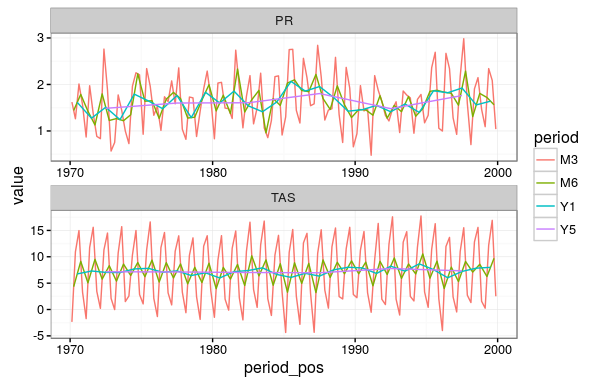Basin average precipitation and temperature at 5 year, 1 year, 6 months and 3 months time scale. Statistical summaries of distribution of each variable at each time scale can be examined in order to compare different data sources (e.g. control simulation to observations) or different time periods (e.g. scenario period to control period). To demonstrate this, let us firts decompose the simulated data for the control and scenario periods in the same way as observations, including also the daily time scale: dobs = decomp(basin_PT$obs_ctrl, period = c('Y5', 'Y1', 'M6', 'M3', 'M1', 'D15', 'D1'))
dctrl = decomp(basin_PT$sim_ctrl, period = c('Y5', 'Y1', 'M6', 'M3', 'M1', 'D15', 'D1')) dscen = decomp(basin_PT$sim_scen, period = c('Y5', 'Y1', 'M6', 'M3', 'M1', 'D15', 'D1'))

The comparison is done with compare function. For instance to compare simulated mean wet-day precipitation and temperature with observation call

bi_bc = compare(x = list(BIAS IN MEAN = dctrl), compare_to = dobs, fun = mean, wet_int_only = TRUE)

with x the list of decomposed variables to be compared to decomposed variables specified by the compare_to argument. The function evaluates distance between statistical characteristics (fun argument) of specified data sets. Distance is measured as difference for variables included in getOption('additive_variables'), i.e. temperature (TAS) by default, and as a ratio for other variables.

The result can be easily visualized by

ggplot(bi_bc[period!='G1']) +
geom_line(aes(x = TS, y = DIF, col = factor(sub_period), group = sub_period)) +
facet_grid(variable~comp, scale = 'free')+
scale_x_log10()+theme_bw()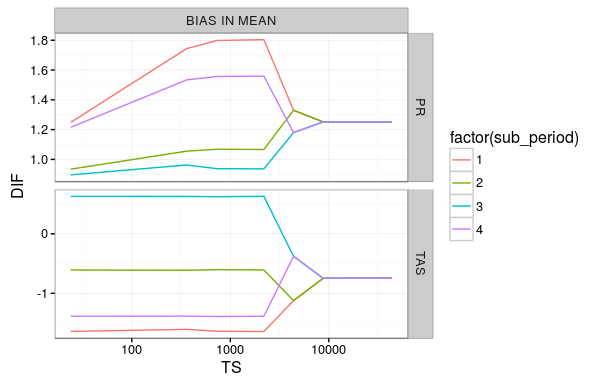Bias in mean basin average precipitation and temperature at various time scales. For sub-seasonal time scales the changes are averaged over seasons.

To compare the 90th quantiles in control and scenario simulations use

bi_dc = compare(x = list(CHANGE IN Q90 = dscen), compare_to = dctrl, fun = Q(.9))

Q is a convenience function provided by the package in order to avoid specification of the 90th quantile as the anonymous function (e.g. fun = function(x)quantile(x, .9)).

Visualization is done in the same way as for bias

ggplot(bi_dc[period!='G1']) +
geom_line(aes(x = TS, y = DIF, col = sscale2sea(sub_period), group = sub_period)) +
facet_grid(variable~comp, scale = 'free')+
scale_x_log10(breaks = tscale(c('Y5', 'Y1', 'M1')), lab = c('Y5', 'Y1', 'M1')) + theme_bw()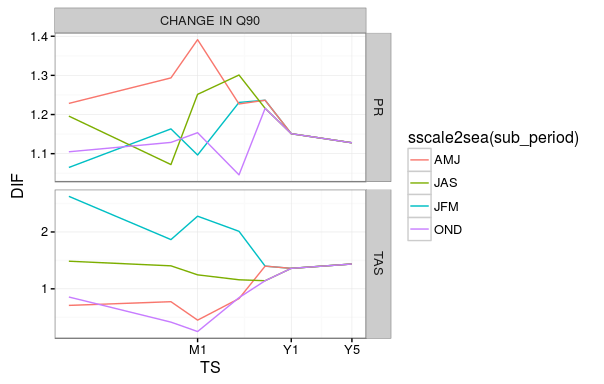Changes in 90th quantile of basin average precipitation and temperature at various time scales. For sub-seasonal time scales the changes are averaged over seasons.

In the call above we used sscale2sea to transform numerical season codes to letters (J - January, F - February etc.) and specified x axis labels and breaks using function tscale converting period codes to hours.

Musica package allows also to compare relations between variables at custom time scales via vcompare function. To assess correlation between precipitation and temperature consider

co = vcompare(x = list(OBS = dobs, CTRL = dctrl, SCEN = dscen), fun = cor)

Visualization is again easy with ggplot2 package:

co = co[, SEA:=sscale2sea(sub_period)]
ggplot(co[period!='G1']) +
geom_line(aes(x = TS, y = value, col = ID))+
facet_grid(VARS~SEA, scales = 'free') +
scale_x_log10(breaks = tscale(c('Y5', 'Y1', 'M1')), lab = c('Y5', 'Y1', 'M1')) +
theme_bw()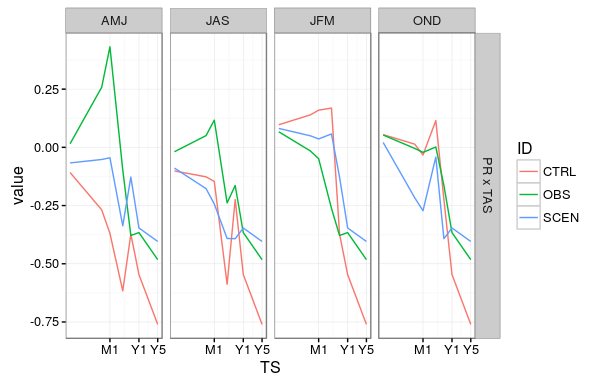Correlation between precipitation and temperature in observed data (green) and control (red) and scenario (blue) simulation

### Multiscale transformations

The transfromations are implemented to work with lists consisting of items FROM, TO and NEWDATA. The transformation is calibrated in order to change variables in FROM to match statistical characteristics of TO. The transformation is then applied to NEWDATA variables. Note, that this concept acoomodate the bias correction as well as the delta change method as indicated in the table below.

FROM TO NEWDATA
bias correction control simulation observed data scenario simulation
delta change control simulation scenario simulation observed data

Considering the basin_PT dataset, the input for the transformation functions can be prepared as

dta4bc = list(FROM = basin_PT$sim_ctrl, TO = basin_PT$obs_ctrl, NEWDATA = basin_PT$sim_scen) for (multiscale) bias correction and dta4dc = list(FROM = basin_PT$sim_ctrl, TO = basin_PT$sim_scen, NEWDATA = basin_PT$obs_ctrl)

for (multiscale) delta change method.

In the case we like to assess the performance of the bias correction we might like to consider

dta4bc0 = list(FROM = basin_PT$sim_ctrl, TO = basin_PT$obs_ctrl, NEWDATA = basin_PT$sim_ctrl) Similarly, to assess the performance of the multiscale delta method we use dta4dc0 = list(FROM = basin_PT$sim_ctrl, TO = basin_PT$sim_scen, NEWDATA = basin_PT$sim_ctrl)

#### Multiscale bias correction

Musica package provides flexible interface for application of bias correction at custom time scale(s), based on the suggestions of Haerter et al. (2011) and Pegram and others (2009). The procedure utilizes standard quantile mapping (see e.g. Gudmundsson et al. 2012) at multiple time scales. Since correction at particular temporal scale influences values at other aggregations, the procedure is applied iterativelly. The procedure is further refered to as multiscale bias correction. Same strategy is adopted also within more complex methods (e.g. Johnson and Sharma 2012; Mehrotra and Sharma 2016).

To apply multiscale bias correction, the function msTrans_abs is used. The function utilizes standard quantile mapping from the qmap-package, but at multiple time scales. Since correction at particular temporal scale influences values at other aggregations, the procedure is applied iterativelly until the maximum number of iterations (specified by maxiter argument) is reached or the difference between succesive iteration step is smaller than tol (1e-4 by default). Differences between corrected and uncorrected variable at longer time scales are used to modify daily values after each iteration step (see e.g. Mehrotra and Sharma 2016; Pegram and others 2009). To make further assessment of the decomposed objects easier, indicator of period within the year (e.g. quarter or month) as specified by agg_by argument is included in the output. Note that the quantile mapping at scales equal or shorter than month are fitted separatelly for each month. The quantile mapping is done at temporal scales specified in period argument.

For instance, standard quantile mapping at daily time step can be performed with

out1 = msTrans_abs(copy(dta4bc0),  maxiter = 10, period = 'D1')

The multiscale correction at daily, monthly, annual and global scale is obtained by

out2 = msTrans_abs(copy(dta4bc0),  maxiter = 10, period = c('G1', 'Y1', 'M1', 'D1'))

To assess the results, first the relevant datasets have to be decomposed:

pers = c('Y1', 'M3' , 'M1', 'D1')
abb = quarter
dobs_0 = decomp(basin_PT$obs_ctrl, period = pers, agg_by = abb) dctrl_0 = decomp(basin_PT$sim_ctrl, period = pers, agg_by = abb)
dQMD1 = decomp(out1, period = pers, agg_by = abb)
dQMMS = decomp(out2, period = pers, agg_by = abb)

The results are compared using the compare function and visualized as demonstrated earlier. For instance the original and residual bias in precipitation and temperature maxima is assessed by

bi_0 = compare(x = list(ORIGINAL = dctrl_0, STANDARD = dQMD1, MULTISCALE = dQMMS), compare_to = dobs_0, fun = max)

ggplot(bi_0[period!='G1']) +
geom_line(aes(x = TS, y = DIF, col = sscale2sea(sub_period), group = sub_period)) +
facet_grid(variable~comp, scale = 'free') +
scale_x_log10(breaks = tscale(c('Y5', 'Y1', 'M1')), lab = c('Y5', 'Y1', 'M1')) + theme_bw()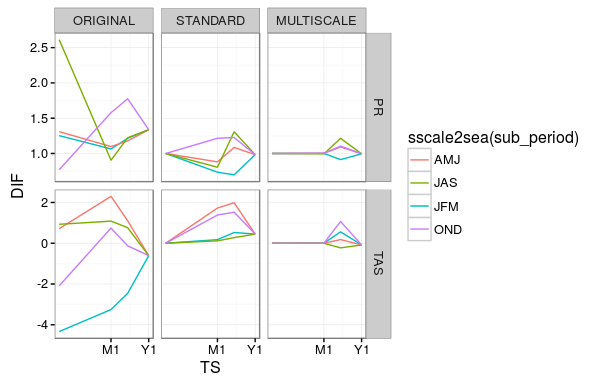Comparison of bias in maximum precipitation and temperature in simulated data and after standard and multiscale bias correction.

#### Multiscale delta method

Let $$F$$ and $$T$$ be the control and scenario simulation, respectively. The method consists in finding a transformation $$f$$ such that

$g_s(T) = g_s[f(F)]$

with $$g_s$$ being a function providing statistical summary at temporal scale(s) $$s$$, most often empirical cumulative distribution function or e.g., mean. In most applications the transformation is determined and applied for each month separately. The pseudocode for the procedure is given in bellow.

input data:
data.frames F, T, N

parameters:
scales      # considered temporal scales
tol         # tolerance
maxiter     # maximum number of iterations
g           # form of the summary function

T* = N

while (error > tol & iter < maxiter){

for (s in scales){

d = dist[g(T), g(F)]
d* = dist[g(T*), g(N)]
T* = update[T*, dist(d, d*)]

}

error = sum_accros_scales(
dist{
dist[g_s(T*), g_s(N)], dist[g_s(T), g_s(F)]
})

iter = iter + 1

}

The input data frames $$F$$ and $$T$$ are used for calibration of the transformation $$f$$, which is then applied to a new data frame $$N$$, resulting in transfromed data frame $$T^*$$. The objective of the procedure is that

$\mathrm{dist}[g_s(T^*), g_s(N)] \sim \mathrm{dist}[g_s(T), g_s(F)], \qquad \textrm{for all} \ s$

with $$\mathrm{dist}$$ the distance, measured as the difference (for temperature) or ratio (for precipitaion). In the procedure, $$T^*$$ is iterativelly updated according to the difference/ratio of $$\mathrm{dist}[g_s(T^*), g_s(N)]$$ and $$\mathrm{dist}[g_s(T), g_s(F)]$$ for each scale $$s$$. The procedure ends when the sum/product of these differences/ratios is sufficiently small or maximum number of iterations is reached. The method is further denoted multiscale delta method.

Musica package currently implements number of choices for $$g_s$$, e.g. mean, empirical distribution function and linear and loess approximation of empirical distribution function.

For instance, standard delta change method at daily time step can be performed with

out3 = msTrans_dif(dta4dc0,  maxiter = 10, period = 'D1', model = 'identity')

to consider changes at global, annual, monthly and daily time scale use

out4 = msTrans_dif(dta4dc0,  maxiter = 10, period = c('G1', 'Y1', 'M1', 'D1'), model = 'identity')

Note, that the model argument specifies the summary function. Standard delta change method considers changes in mean (model = "const"). Here we assess the changes in the whole distribution function.

To assess the results, first the relevant datasets have to be decomposed:

pers = c('Y1', 'M3' , 'M1', 'D1')
abb = quarter
dctrl_0 = decomp(basin_PT$sim_ctrl, period = pers, agg_by = abb) dscen_0 = decomp(basin_PT$sim_scen, period = pers, agg_by = abb)

dDCD1 = decomp(out3, period = pers, agg_by = abb)
dDCMS = decomp(out4, period = pers, agg_by = abb)

The results are compared using the compare function.

bi_1 = compare(x = list(SIMULATED = dscen_0, STANDARD = dDCD1, MULTISCALE = dDCMS), compare_to = dctrl_0, fun = max)

ggplot(bi_1[period!='G1']) +
geom_line(aes(x = TS, y = DIF, col = sscale2sea(sub_period), group = sub_period)) +
facet_grid(variable~comp, scale = 'free') +
scale_x_log10(breaks = tscale(c('Y5', 'Y1', 'M1')), lab = c('Y5', 'Y1', 'M1')) + theme_bw()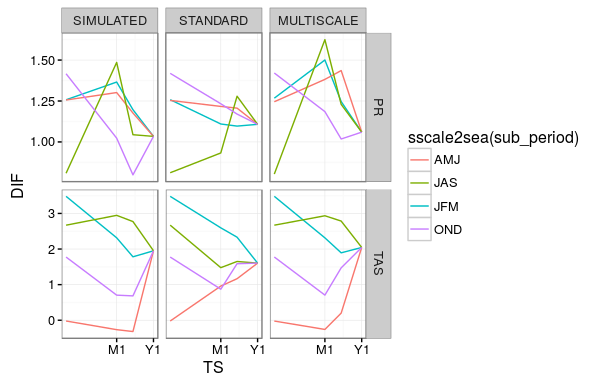Comparison of changes in maximum precipitation and temperature in simulated data and after standard and multiscale delta change transformation.